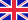•# Order theory

Autor: Source: Wikipedia

Source: Wikipedia. Pages: 121. Chapters: Zorn's lemma, Well-order, Total order, Interval, Supremum, Ordered pair, Dedekind cut, Infimum, Ultrafilter, Monotonic function, Directed set, Möbius inversion formula, Cofinality, Upper and lower bounds, Embedding,... Viac o knihe

Na objednávku, dodanie 2-4 týždne

25.33 €

bežná cena: 29.80 €

## O knihe

Source: Wikipedia. Pages: 121. Chapters: Zorn's lemma, Well-order, Total order, Interval, Supremum, Ordered pair, Dedekind cut, Infimum, Ultrafilter, Monotonic function, Directed set, Möbius inversion formula, Cofinality, Upper and lower bounds, Embedding, Ascending chain condition, Incidence algebra, Bounded set, Hausdorff maximal principle, Preorder, Infinite descending chain, Partially ordered set, Galois connection, Partially-ordered group, Causal sets, Glossary of order theory, Ordinal optimization, Completeness, Closure operator, Series-parallel partial order, Stone duality, 1/3-2/3 conjecture, Alexandrov topology, Dilworth's theorem, Order topology, Boolean prime ideal theorem, Semiorder, Strict weak ordering, Hasse diagram, Infinite divisibility, Knaster-Tarski theorem, Comparability graph, Lexicographical order, Graded poset, Greedoid, Well-quasi-ordering, Complete Boolean algebra, Maximal element, Monomial order, Limit-preserving function, Specializationorder, Linear continuum, Majorization, Linear extension, Complete Heyting algebra, Join and meet, Complete partial order, Order dimension, Residuated mapping, Compact element, Kruskal's tree theorem, Fence, Scott domain, Distributivity, Scott continuity, Supermodular function, Completely distributive lattice, Prewellordering, Order-embedding, Multitree, Antichain, Topkis's theorem, Greatest element, List of order theory topics, Suslin's problem, Upper set, Bourbaki-Witt theorem, Covering relation, Lubell-Yamamoto-Meshalkin inequality, Critical pair, Bounded complete poset, Quantale, Schnyder's theorem, Atom, Countable chain condition, Upper topology, Disjunction property of Wallman, Dickson's lemma, Order isomorphism, Least fixed point, Containment order, Preordered class, Trichotomy, Kleene-Brouwer order, Bound graph, Sperner property of a partially ordered set, Poset topology, Kleene fixed-point theorem, Pseudoideal, Schur-Horn theorem, Frink ideal, Interval order, Chain complete, Higman's lemma, Ranked poset, Countryman line, Pasch's theorem, Locally finite poset, Product order, Scattered order, Aronszajn line, Strong antichain, Dense order, Fréchet filter, Tukey's lemma, Shortlex order. Excerpt: The causal sets programme is an approach to quantum gravity. Its founding principle is that spacetime is fundamentally discrete and that the spacetime events are related by a partial order. This partial order has the physical meaning of the causality relations between spacetime events. The programme is based on a theorem by David Malament which states that if there is a bijective map between two past and future distinguishing spacetimes which preserves their causal structure then the map is a conformal isomorphism. The conformal factor that is left undetermined is related to the volume of regions in the spacetime. This volume factor can be recovered by specifying a volume element for each spacetime point. The volume of a spacetime region could then be found by counting the number of points in that region. Causal sets was initiated by Rafael Sorkin who continues to be the main proponent of the programme. He has coined the slogan "Order + Number = Geometry" to characterise the above argument. The programme provides a theory in which spacetime is fundamentally discrete while retaining local Lorentz invariance. A causal set (or causet) is a set with a partial order relation which is Here card() denotes the cardinality of a set . We'll write if and . The set represents the set of spaceti...

• Vydavateľstvo: Books LLC, Reference Series
• Rok vydania: 2011
• Formát: Paperback
• Rozmer: 246 x 189 mm
• Jazyk: Anglický jazyk
• ISBN: 9781157490739

Generuje redakčný systém BUXUS CMS spoločnosti ui42.